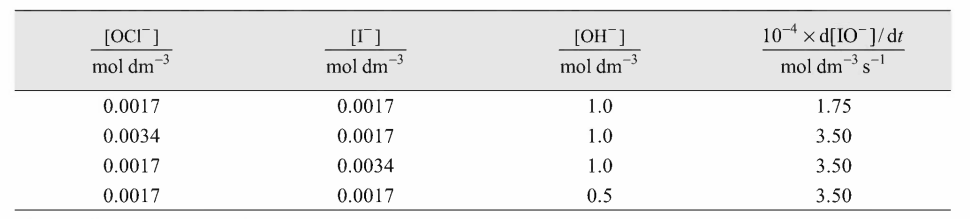The kinetic data for the reaction OCl−+I−⟶OH−OI−+Cl− at 298 is as follows:The rate low for the formation of OI− is

# The kinetic data for the reaction ${\mathrm{OCl}}^{-}+{\mathrm{I}}^{-}\stackrel{{\mathrm{OH}}^{-}}{⟶}{\mathrm{OI}}^{-}+{\mathrm{Cl}}^{-}$ at 298 is as follows:The rate low for the formation of ${\mathrm{OI}}^{-}$ is

1. A

$r=k\left[{\mathrm{OCl}}^{-}\right]\left[{\mathrm{I}}^{-}\right]$

2. B

$r=k{\left[{\mathrm{OCl}}^{-}\right]}^{2}$

3. C

$r=k{\left[{\mathrm{I}}^{-}\right]}^{2}\left[{\mathrm{OCl}}^{-}\right]$

4. D

$r=k\left[{\mathrm{OCl}}^{-}\right]\left[{\mathrm{I}}^{-}\right]/\left[{\mathrm{OH}}^{-}\right]$

Fill Out the Form for Expert Academic Guidance!l

+91

Live ClassesBooksTest SeriesSelf Learning

Verify OTP Code (required)

### Solution:

On doubling the $\left[{\mathrm{OCl}}^{-}\right]$ keeping the $\left[{\mathrm{I}}^{-}\right]$and $\left[{\mathrm{OH}}^{-}\right]$ constant, the rate of formation of $\left[{\mathrm{OH}}^{-}\right]$ is doubled, hence the order of reaction with respect to $\left[{\mathrm{OCl}}^{-}\right]$ is one. On doubling the $\left[{\mathrm{I}}^{-}\right]$ keeping the $\left[{\mathrm{OCl}}^{-}\right]$ and $\left[{\mathrm{OH}}^{-}\right]$ constant, the rate of formation of $\left[{\mathrm{OI}}^{-}\right]$ is doubled, hence the order of reaction with respect to 1- is one. On changing the $\left[{\mathrm{OH}}^{-}\right]$ to a half-value, the rate of formation of $\left[{\mathrm{OI}}^{-}\right]$ is doubled. Hence, the order of reaction with respect to $\left[{\mathrm{OH}}^{-}\right]$ is -1.

Hence the rate law is $r=k\left[{\mathrm{OCl}}^{-}\right]\left[{\mathrm{I}}^{-}\right]{\left[{\mathrm{OH}}^{-}\right]}^{-1}=k\left[{\mathrm{OCl}}^{-}\right]\left[{\mathrm{I}}^{-}\right]/\left[{\mathrm{OH}}^{-}\right]$+91

Live ClassesBooksTest SeriesSelf Learning

Verify OTP Code (required)